# GMAT Math : DSQ: Calculating the length of the side of an acute / obtuse triangle

## Example Questions

### Example Question #1 : Dsq: Calculating The Length Of The Side Of An Acute / Obtuse Triangle

Is the triangle isosceles?

Statement 1: The triangle has vertices A(1,5), B(4,2), and C(5,6).

Statement 2: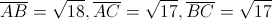BOTH statements TOGETHER are sufficient, but NEITHER statement ALONE is sufficient.

EACH statement ALONE is sufficient.

Statement 2 ALONE is sufficient, but statement 1 is not sufficient.

Statement 1 ALONE is sufficient, but statement 2 is not sufficient.

Statements 1 and 2 TOGETHER are NOT sufficient.

EACH statement ALONE is sufficient.

Explanation:

For a triangle to be isosceles, two of the sides must be equal.  To determine wheter this is true, we must have the three side lengths.  Statement 2 gives us those three side lengths.  However, Statement 1 also gives us all of the information we need by giving us the three vertices.  By using the distance formula, we can easily get the three triangle sides from the vertices.  Therefore both statements alone are sufficient.

### Example Question #2 : Dsq: Calculating The Length Of The Side Of An Acute / Obtuse Triangle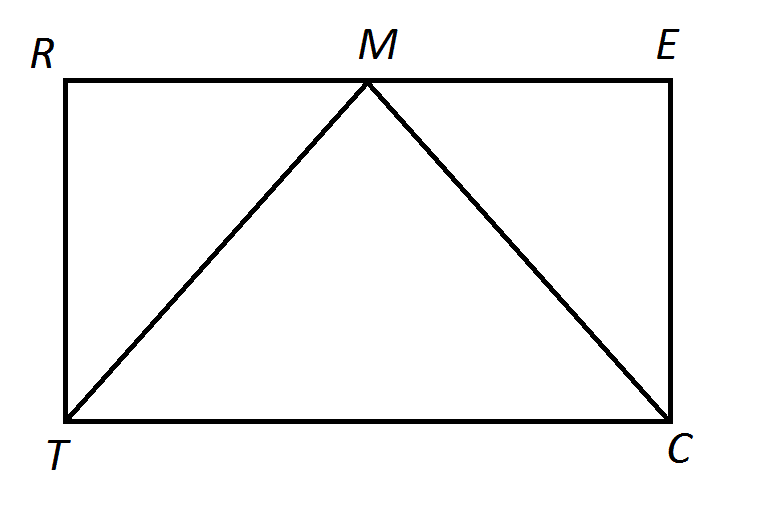Note: Figure NOT drawn to scale.

The above shows a triangle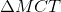inscribed inside a rectangle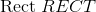. isisosceles?

Statement 1:is the midpoint of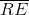.

Statement 2: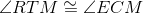BOTH statements TOGETHER are insufficient to answer the question.

Statement 2 ALONE is sufficient to answer the question, but Statement 1 ALONE is NOT sufficient to answer the question.

BOTH statements TOGETHER are sufficient to answer the question, but NEITHER statement ALONE is sufficient to answer the question.

Statement 1 ALONE is sufficient to answer the question, but Statement 2 ALONE is NOT sufficient to answer the question.

EITHER statement ALONE is sufficient to answer the question.

EITHER statement ALONE is sufficient to answer the question.

Explanation:

We show Statement 1 alone is sufficient:

Ifis the midpoint of, then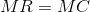. Opposite sides of a rectangle are congruent, so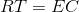; all angles of a rectangle, being right angles, are congruent, so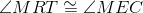. This sets up the conditions for the Side-Angle-Side Theorem, and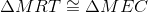. Consequently,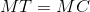, andis isosceles.

Now, we show Statement 2 alone is sufficient:

If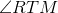, and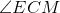are congruent, then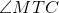and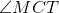, being complements of congruent angles, are congruent themselves. By the Isosceles Triangle Theorem,is isosceles.

### Example Question #3 : Dsq: Calculating The Length Of The Side Of An Acute / Obtuse Triangle

Which side of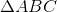is the longest?

Statement 1: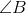is an obtuse angle.

Statement 2: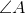and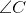are both acute angles.

Statement 2 ALONE is sufficient to answer the question, but Statement 1 ALONE is NOT sufficient to answer the question.

BOTH statements TOGETHER are sufficient to answer the question, but NEITHER statement ALONE is sufficient to answer the question.

Statement 1 ALONE is sufficient to answer the question, but Statement 2 ALONE is NOT sufficient to answer the question.

EITHER statement ALONE is sufficient to answer the question.

BOTH statements TOGETHER are insufficient to answer the question.

Statement 1 ALONE is sufficient to answer the question, but Statement 2 ALONE is NOT sufficient to answer the question.

Explanation:

If we only know that two interior angles of a triangle are acute, we cannot deduce the measure of the third, or even if it is obtuse or right; therefore, Statement 2 alone does not help us.

If we know thatis an obtuse angle, however, we can deduce thatandare both acute angles, since at least two interior angles of a triangle are acute. Therefore, we can deduce thathas the greatest measure, and that its opposite side,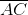,  is the longest.

### Example Question #4 : Dsq: Calculating The Length Of The Side Of An Acute / Obtuse Triangle

Isisosceles?

Statement 1: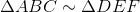Statement 2: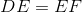Statement 2 ALONE is sufficient to answer the question, but Statement 1 ALONE is NOT sufficient to answer the question.

Statement 1 ALONE is sufficient to answer the question, but Statement 2 ALONE is NOT sufficient to answer the question.

EITHER statement ALONE is sufficient to answer the question.

BOTH statements TOGETHER are sufficient to answer the question, but NEITHER statement ALONE is sufficient to answer the question.

BOTH statements TOGETHER are insufficient to answer the question.

BOTH statements TOGETHER are sufficient to answer the question, but NEITHER statement ALONE is sufficient to answer the question.

Explanation:

Statement 1 alone does not tell us anything unless we know the relative lengths of the sides of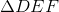; Statement 2 only gives us information about another triangle.

Suppose we assume both statements. Then by similarity,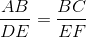.

Since, then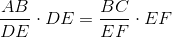, or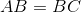This makesisosceles.

### Example Question #5 : Dsq: Calculating The Length Of The Side Of An Acute / Obtuse Triangle

Which of the three sides ofis the longest?

Statement 1: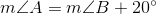Statement 2: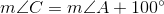BOTH statements TOGETHER are sufficient to answer the question, but NEITHER statement ALONE is sufficient to answer the question.

Statement 2 ALONE is sufficient to answer the question, but Statement 1 ALONE is NOT sufficient to answer the question.

EITHER statement ALONE is sufficient to answer the question.

Statement 1 ALONE is sufficient to answer the question, but Statement 2 ALONE is NOT sufficient to answer the question.

BOTH statements TOGETHER are insufficient to answer the question.

Statement 2 ALONE is sufficient to answer the question, but Statement 1 ALONE is NOT sufficient to answer the question.

Explanation:

The longest side of a triangle is opposite the angle of greatest measure.

From Statement 1 alone, we can find two possible scenarios with different answers:

Case 1: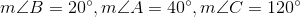Case 2: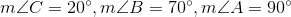In both cases,, but in Case 1,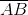is the longest side, and in Case 2,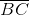is the longest side.

From Statement 2 alone, however, we know that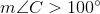, sois obtuse and the other two angles are acute. That makesthe longest side.

### Example Question #6 : Dsq: Calculating The Length Of The Side Of An Acute / Obtuse Triangle

True or false:is scalene.

Statement 1: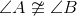Statement 2: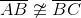Statement 2 ALONE is sufficient to answer the question, but Statement 1 ALONE is NOT sufficient to answer the question.

BOTH statements TOGETHER are sufficient to answer the question, but NEITHER statement ALONE is sufficient to answer the question.

EITHER statement ALONE is sufficient to answer the question.

BOTH statements TOGETHER are insufficient to answer the question.

Statement 1 ALONE is sufficient to answer the question, but Statement 2 ALONE is NOT sufficient to answer the question.

BOTH statements TOGETHER are insufficient to answer the question.

Explanation:

Assume both statements are true.

By definition, a scalene triangle has three noncongruent sides. Sides opposite noncongruent angles of a triangle are noncongruent, so as a consequence of Statement 1,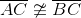. Statement 2 alone establishes that. However, the two statements together do not establish whether or not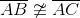, so it is not clear whetheris scalene or isosceles.

### Example Question #7 : Dsq: Calculating The Length Of The Side Of An Acute / Obtuse Triangle

True or false:is scalene.

Statement 1:Statement 2: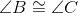BOTH statements TOGETHER are sufficient to answer the question, but NEITHER statement ALONE is sufficient to answer the question.

Statement 2 ALONE is sufficient to answer the question, but Statement 1 ALONE is NOT sufficient to answer the question.

EITHER statement ALONE is sufficient to answer the question.

Statement 1 ALONE is sufficient to answer the question, but Statement 2 ALONE is NOT sufficient to answer the question.

BOTH statements TOGETHER are insufficient to answer the question.

Statement 2 ALONE is sufficient to answer the question, but Statement 1 ALONE is NOT sufficient to answer the question.

Explanation:

By definition, a scalene triangle has three noncongruent sides.

Statement 1 alone states that two sides are noncongruent, but no information is given about whether or not third sideis congruent to either of the other sides.

Assume Statement 2 alone. In a triangle, sides opposite congruent angles are congruent, so it follows that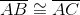. The triangle cannot be scalene.

### Example Question #8 : Dsq: Calculating The Length Of The Side Of An Acute / Obtuse Triangle

True or false:is scalene.

Statement 1: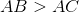Statement 2: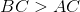BOTH statements TOGETHER are insufficient to answer the question.

Statement 2 ALONE is sufficient to answer the question, but Statement 1 ALONE is NOT sufficient to answer the question.

Statement 1 ALONE is sufficient to answer the question, but Statement 2 ALONE is NOT sufficient to answer the question.

BOTH statements TOGETHER are sufficient to answer the question, but NEITHER statement ALONE is sufficient to answer the question.

EITHER statement ALONE is sufficient to answer the question.

BOTH statements TOGETHER are insufficient to answer the question.

Explanation:

By definition, a scalene triangle has three noncongruent sides.

If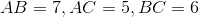, thenand, and the triangle is scalene.

If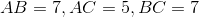, thenand, but, so the triangle is not scalene.

The two statements together are insufficient.

Tired of practice problems?

Try live online GMAT prep today.